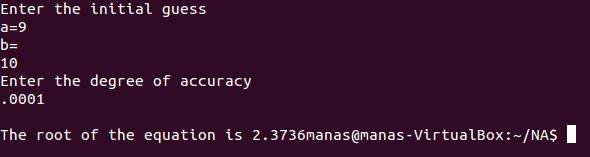# C++ Program for Secant Method to find the roots of an Equation

```//Secant Method for finding the roots of an equation
#include<iostream>
#include<iomanip>
#include<cmath>
using namespace std;
double f(double x);    //declare the function for the given equation
double f(double x)    //define the function here, ie give the equation
{
double a=pow(x,3)-x-11.0;    //write the equation whose roots are to be determined
return a;
}
int main()
{
cout.precision(4);
cout.setf(ios::fixed);        //set the precision of the output
double a,b,c,e;
cout<<"Enter the initial guess\na=";
cin>>b;
cout<<"b=\n";                //take an intial guess
cin>>c;
cout<<"Enter the degree of accuracy\n";
cin>>e;                    //take the desired accuracy
do
{
a=b;
b=c;                //make b equal to the last calculated value of c
c=b-(b-a)/(f(b)-f(a))*f(b);    //calculate c
if (f(c)==0)
{
cout<<"\nThe root of the equation is "<<c;    //print the root
return 0;
}
}while(abs(c-b)>=e);            //check if the error is greater than the desired accuracy
cout<<"\nThe root of the equation is "<<c;    //print the root
return 0;
}

```Explanation of the code:

[wpedon id="7041" align="center"]

## 4 thoughts on “C++ Program for Secant Method to find the roots of an Equation”

1. how can i get PDF including some of this numerical problem with solution??

2. the code given is differebt from the one used in the video

This site uses Akismet to reduce spam. Learn how your comment data is processed.